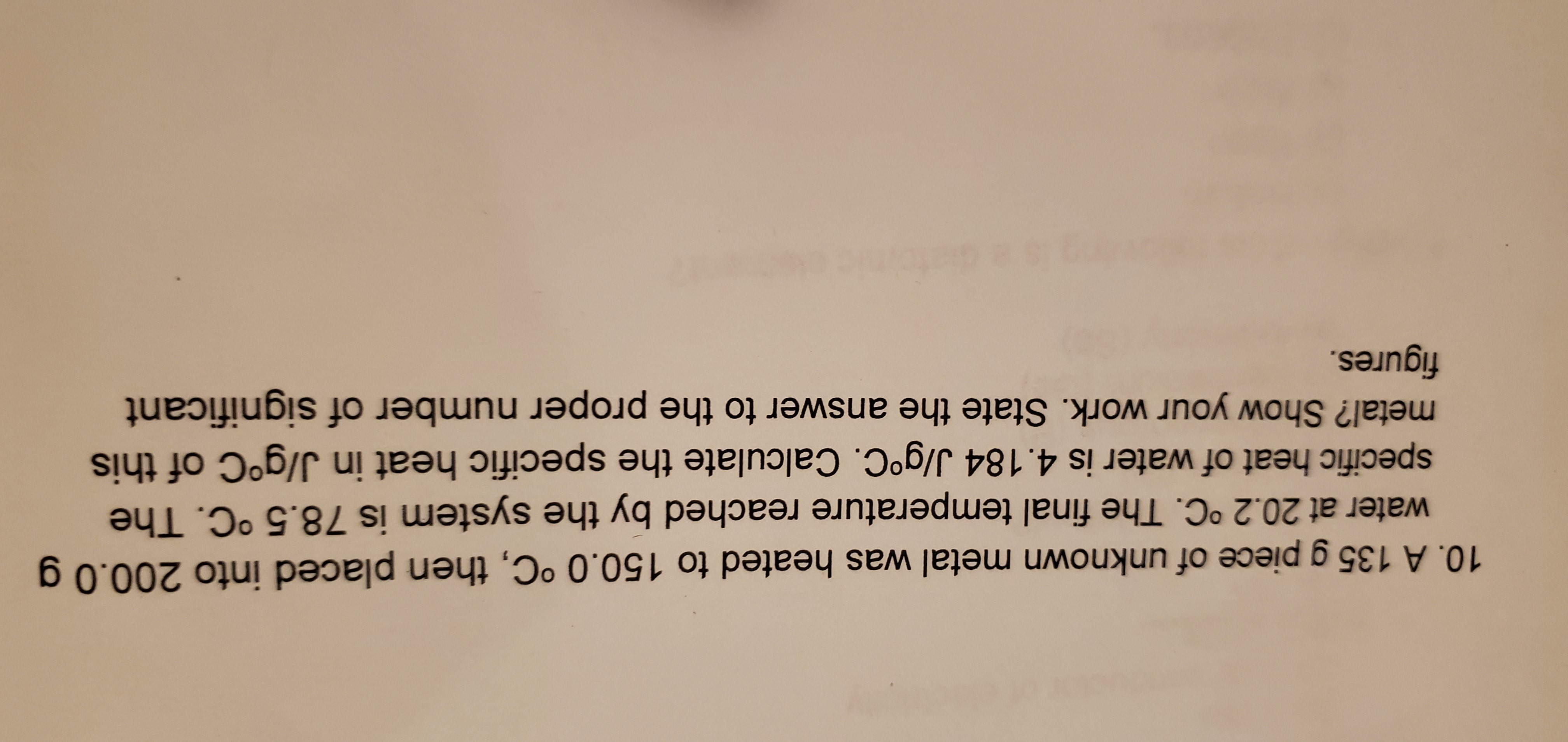# 10. A 135 g piece of unknown metal was heated to 150.0 °C, then placed into 200.0 g water at 20.2 °C. The final temperature reached by the system is 78.5 °C. The specific heat of water is 4.184 J/g°C. Calculate the specific heat in J/g°C of this metal? Show your work. State the answer to the proper number of significant figures.

Questionhelp_outlineImage Transcriptionclose10. A 135 g piece of unknown metal was heated to 150.0 °C, then placed into 200.0 g water at 20.2 °C. The final temperature reached by the system is 78.5 °C. The specific heat of water is 4.184 J/g°C. Calculate the specific heat in J/g°C of this metal? Show your work. State the answer to the proper number of significant figures. fullscreen

### Want to see the step-by-step answer?